We advise you to have seen the Melo-rhythm and the Melo-harmony sections
before seeing the Melo-lines.
They are a necessary prerequisite.

Here again, as with all generations, we will find
Basic Materials and Transformations.

Basic Materials

The most important element of the Basic Materials of Melo-lines
is the priority of the Orbit 1 - Orbit 1 line.
. The most fundamental melody would have only Orbit 1, from beginning to end.
Believe it or not, examples exist of this (the "A" sections of Au clair de la lune).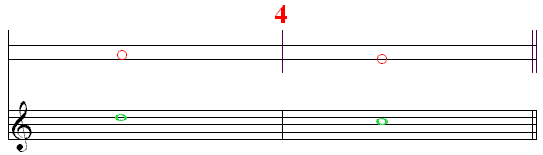As was the case for the Basic Materials of Melo-rhythm and Melo-harmony,
we start with the Fundamental Cell, the very last one.
It will be attributed the Basic Melo-line which is the Orbit 1 - Orbit 1 line
on the Melo-harmony Fundamental cell M4 DOMINANT / TONIC, and
we will have the notes D / C, in the usual key of C major.
This basic cell is the most common ending in melodies.

TransformationsThe first form of Transformation is one in which the off-beat of this basic Melo-line can be changed -
1. To Orbit 2 which "substitutes" for the original Orbit 1
producing the Orbit 2 - Orbit 1 Melo-line.
On the Melo-harmony Fundamental cell M4, we will have the notes B / C,
which are found in several melodies.
2. To Orbit 0 which "substitutes" for the original Orbit 1
producing the Orbit 0 - Orbit 1 Melo-line.
On the Melo-harmony Fundamental cell M4, we will have the notes G / C,
the Fundamental Bass,
which are found in a few melodies.

It is interesting to note that the Orbit priorities are not the same as they were for Harmony -
where the Orbit 3 - Orbit 2 line was the most prominent,
followed by the Orbit 2 - Orbit 1 line,
the resolutions of the two Secondary Notes.
In Melody, the Orbit 1 - Orbit 1 is the prominent line
with the Orbit 2 - Orbit 1 line substituting for it, in a secondary role.
The Orbit 0 - Orbit 1 line is less prominent still
used almost exclusively when several lines are combined.

Two Cells

If we open the Fundamental Melo-harmonic cell M4 DOMINANT / TONIC -
the chord pattern will become M34 TONIC / DOMINANT \ DOMINANT / TONIC.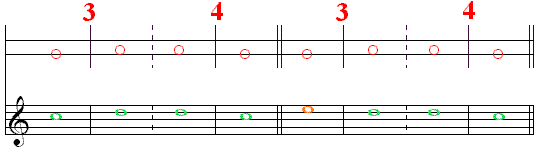The Basic Fundamental Melo-line Cell Orbit 1 - Orbit 1, with the notes D / C,
can be preceded by either Orbit 1 or Orbit 2
on the TONIC chord at the beginning
for which we have the notes C / D \ D / C, or E / D \ D / C.
We keep the same orbit on the two DOMINANT chords.
The C / D \ D / C line is the totally Orbit 1 line of which we spoke a little earlier.
It is not seen as often as the E / D \ D / C line which has more Orbit variety.

Symmetry
Note the inverse symmetry in the C / D \ D / C line, and
the direct symmetry in the E / D \ D / C line.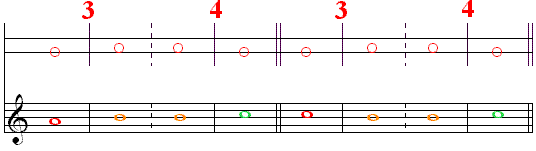The first Transformation Melo-line Cell Orbit 2 - Orbit 1, with the notes B / C,
can be preceded by either Orbit 3 itself or by its substitution Orbit 1
(colored here like the original Orbit 3)
always on the TONIC chord at the beginning,
for which we have the notes A / B \ B / C, or C / B \ B / C.
We keep the same orbit on the two DOMINANT chords.
The C / B \ B / C line is used more often than the A / B \ B / C,
Melody prefering Orbit 1 to Orbit 3.

Symmetry
Note the inverse symmetry in the C / B \ B / C line, and
the direct symmetry in the A / B \ B / C line.

The second Transformation Melo-line Cell Orbit 0 - Orbit 1, with the notes G / C,
is seldom used by itself, and we will return to it when we combine several lines.

Several Lines

In the preceding examples, we can take advantage of the repeated DOMINANT chords to combine several lines
at first only two lines, and eventually three.
The chord pattern remains M34 TONIC / DOMINANT \ DOMINANT / TONIC.

Lines 1-1 and 2-1
Orbit 1 will be placed on one DOMINANT chord and Orbit 2 on the other.
Both orbits on the DOMINANT chord will resolve to Orbit 1 on the final TONIC chord.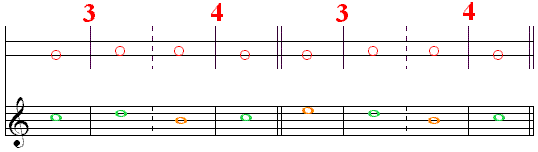On the opening TONIC chord, we find -
Orbit 1, the note C, in the first example, and
Orbit 2, the note E, in the second example.
In both examples, we find -
Orbit 1, the note D, on the first DOMINANT chord and
Orbit 2, the note B, on the second DOMINANT chord,
which both resolve to Orbit 1, the note C, on the final TONIC chord.
These examples will be evaluated a little later,
some more valid than others.

Symmetry
Note the inverse symmetry in the E / D \ B / C line, and
the direct symmetry in the C / D \ B / C line.On the opening TONIC chord, we find -
Orbit 1, the note C, (substituting for Orbit 3) in the first example, and
Orbit 3, the note A, in the second example.
In the both examples, we find -
Orbit 2, the note B, on the first DOMINANT chord and
Orbit 1, the note D, on the second DOMINANT chord,
which both resolve to Orbit 1, the note C, on the final TONIC chord.

Symmetry
Note the inverse symmetry in the A / B \ D / C line, and
the direct symmetry in the C / B \ D / C line.

Adding Line 0-1
Orbit 0 will be placed on the second DOMINANT chord and
either Orbit 1 or Orbit 2 on the first.
Both orbits on the DOMINANT chord will resolve to Orbit 1 on the final TONIC chord.On the opening TONIC chord, we find -
Orbit 1, the note C, in the first example, and
Orbit 2, the note E, in the second example.
In the both examples, we find -
Orbit 1, the note D, on the first DOMINANT chord and
Orbit 0, the note G, on the second DOMINANT chord,
which both resolve to Orbit 1, the note C, on the final TONIC chord.On the opening TONIC chord, we find -
Orbit 1, the note C, (substituting for Orbit 3) in the first example, and
Orbit 3, the note A, in the second example.
In the both examples, we find -
Orbit 2, the note B, on the first DOMINANT chord and
Orbit 0, the note G, on the second DOMINANT chord,
which both resolve to Orbit 1, the note C, on the final TONIC chord.
Orbit 0, the note G, is often placed an octave higher.

Combining Three Lines
In order to have enough space ("box-compartments") to place the three lines,
we will subdivide the two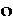on the DOMINANT chords into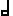.On the opening TONIC chord, we find -
Orbit 1, the note C, in the first example, and
Orbit 2, the note E, in the second example.
On the two DOMINANT chords, we find -
Orbit 1, Orbit 2, Orbit 0, Orbit 1, the notes D / B \ G / D, in the first example, and
Orbit 1, Orbit 0, Orbit 1, Orbit 2, the notes D / G \ D / B, in the second example,
which all resolve to Orbit 1, the note C, on the final TONIC chord.On the opening TONIC chord, we find -
Orbit 1, the note C, (substituting for Orbit 3) in the first example, and
Orbit 3, the note A, in the second example.
On the two DOMINANT chords, we find -
Orbit 2, Orbit 1, Orbit 0, Orbit 2, the notes B / D \ G / B, in the first example, and
Orbit 2, Orbit 0, Orbit 2, Orbit 1, the notes B / G \ B / D, in the second example,
which all resolve to Orbit 1, the note C, on the final TONIC chord.

These combinations of three lines evidently give the melody an "arpeggio" flavor.

The possibilities become more numerous as we are offered more choices.

Non-chordal Tones

Adding Non-chordal Tones will evidently multiply the possibilities far beyond our capacity of listing them -
each Non-chordal Tone offering the possibility of superior or inferior
possibly both, as we will soon see.
These "Non-chordal Tones" may, or may not be, incorporated into the harmony of the accompaniment.
When they are NOT incorporated into the harmony they are often called "Non-harmonic Tones"
which seems a very good idea.
The chord pattern remains M34 TONIC / DOMINANT \ DOMINANT / TONIC,
and we will continue our variations on theof the two DOMINANT chords.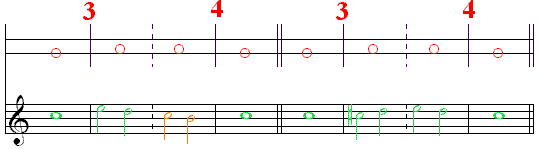On the opening TONIC chord, we find -
Orbit 1, the note C, in both examples.
On the two DOMINANT chords, we find -
sus6 and Orbit 1 itself, sus4 and Orbit 2 itself, the notes E - D - C - B, in the first example, and
push+4 and Orbit 1 itself, sus 6 and Orbit 1 itself, the notes C# - D - E - D, in the second example,
which all resolve to Orbit 1, the note C, on the final TONIC chord.
The first example is simpler in Non-chordal Tones (both descending)
and more complex in lines (combining two).
The second example is more complex in Non-chordal Tones (one chromatic ascending, one descending)
and simpler in lines (only one).On the opening TONIC chord, we find -
Orbit 3, the note A, in the first example, and
Orbit 1, the note C, (substituting for Orbit 3) in the second example.
On the two DOMINANT chords, we find -
sus4 and Orbit 2 itself, sus6 and Orbit 1 itself, the notes C - B - E - D, in the first example, and
sus4 and Orbit 2 itself, push2 and Orbit 2 itself, the notes C - B - A - B, in the second example,
which all resolve to Orbit 1, the note C, on the final TONIC chord.
Here again, the first example is simpler in Non-chordal Tones (both descending)
and more complex in lines (combining two) and
the second example is more complex in Non-chordal Tones (one descending, one ascending)
and simpler in lines (only one).

With the Three Lines
It is possible to place a Non-chordal Tone with the three lines.On the opening TONIC chord, we find -
Orbit 1, the note C, in the first example, and
Orbit 2, the note E, in the second example.
On the two DOMINANT chords, we find -
Orbit 1, Orbit 0, push2 and Orbit 2 itself, the notes D - G - A - B, in the first example, and
Orbit 1, sus4 and Orbit 2 itself, Orbit 0, the notes D - C - B - G, in the second example,
which all resolve to Orbit 1, the note C, on the final TONIC chord.On the opening TONIC chord, we find -
Orbit 2, the note E, in the first example.
Orbit 1, the note C, (substituting for Orbit 3) in the second example.
On the two DOMINANT chords, we find -
Orbit 1, push+7 and Orbit 0 itself, Orbit 2, the notes D - F# - G - B, in the first example, and
Orbit 2, Orbit 0, sus6 and Orbit 1 itself, the notes B - G - E - D, in the second example,
which all resolve to Orbit 1, the note C, on the final TONIC chord.

Two Non-chordal Tones in a Row
It is possible to place two successive Non-chordal Tones within a given line.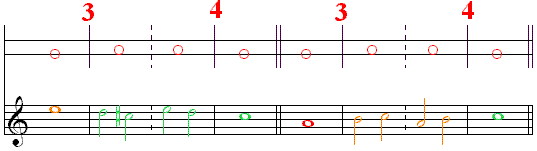On the opening TONIC chord, we find -
Orbit 2, the note E, in the first example.
Orbit 3, the note A, in the second example.
On the two DOMINANT chords, we find -
Orbit 1, push+4, sus6, and Orbit 1 itself, the notes D - C# - E - D, in the first example, and
Orbit 2, sus4, push2, and Orbit 2 itself, the notes B - C - A - B, in the second example,
which all resolve to Orbit 1, the note C, on the final TONIC chord.
These are occasionally called "double embroideries".

Double Non-chordal Tones
It is possible to place Double Non-chordal Tones within a given line.On the opening TONIC chord, we find -
Orbit 1, the note C, in the first example.
Orbit 0, the note G, in the second example.
On the two DOMINANT chords, we find -
Orbit 1, sus-sus7, sus6, and Orbit 1 itself, the notes D - F - E - D, in the first example, and
Orbit 0, sus-sus7, sus6, and Orbit 1 itself, the notes G - F - E - D, in the second example,
which all resolve to Orbit 1, the note C, on the final TONIC chord.
The second example is so popular that we affectionately call it "the winner".

Other Chord Patterns

All the generative procedures applied above could be repeated on different chord patterns.
We have chosen a few of the most popular and presented them with some examples.
Since the closing M4 DOMINANT / TONIC is always the same
we only present new choices in the first cell.

The 64 Pattern
M64 is composed of COUNTER / TONIC \ DOMINANT / TONIC
The is a Metamorphosis 1 on the first TONIC which inverses Orbit 0 and Orbit 1.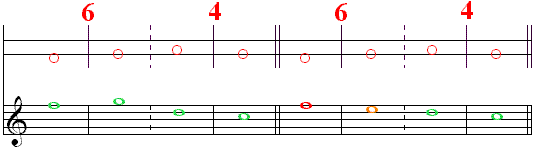In the first example, we find -
Orbit 1, the note F, on the COUNTER chord,
Orbit 1, the note G (which becomes Orbit 0), on the TONIC chord,
Orbit 1, the note D, on the DOMINANT chord, and
Orbit 1, the note C, on the TONIC chord,
in all, the notes F / G \ D / C.
Since each note is on a different chord, it immediately resolves to the following chord.
In the second example, we find -
Orbit 1, the note F, (which substitutes for Orbit 3) on the COUNTER chord,
(and is here colored accordingly)
Orbit 2, the note E, on the TONIC chord,
Orbit 1, the note D, on the DOMINANT chord, and
Orbit 1, the note C, on the TONIC chord,
in all, the notes F / E \ D / C.
The second example is a slightly shorter version of our "winner".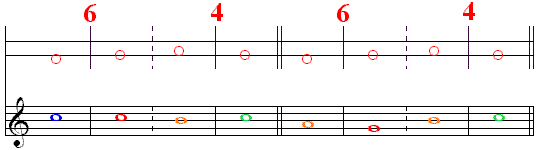In the first example, we find -
Orbit 0, the note C, on the COUNTER chord,
Orbit 0, the note C (which substitutes for Orbit 3), on the TONIC chord,
Orbit 2, the note B, on the DOMINANT chord, and
Orbit 1, the note C, on the TONIC chord,
in all, the notes C / C \ B / C.
In the second example, we find -
Orbit 2, the note A, on the COUNTER chord,
Orbit 1, the note G (which becomes Orbit 0 and substitutes for Orbit 3), on the TONIC chord,
Orbit 2, the note B, on the DOMINANT chord, and
Orbit 1, the note C, on the TONIC chord.
in all, the notes A / G \ B / C.
The second example is a Mozart favorite.

The 74 Pattern
M74 is composed of TONIC / COUNTER \ DOMINANT / TONIC
There is a Metamorphosis 4 on the COUNTER (inversing 0-3 and 1-2)
and a Metamorphosis 1 on the DOMINANT (inversing 0-1).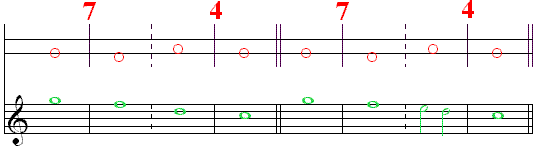In the first example, we find -
Orbit 1, the note G, on the TONIC chord,
Orbit 1, the note F (which becomes a double Non-chordal Tone of Orbit 0), on the COUNTER chord,
Orbit 1, the note D, on the DOMINANT chord, and
Orbit 1, the note C, on the TONIC chord,
in all, the notes G / F \ D / C.
In the second example, we find -
Orbit 1, the note G, on the TONIC chord,
Orbit 1, the note F (which becomes a double Non-chordal Tone of Orbit 0), on the COUNTER chord,
sus6 and Orbit 1 itself, the notes E - D, on the DOMINANT chord, and
Orbit 1, the note C, on the TONIC chord,
in all, the notes G / F \ E-D / C.
The second example is another version of "the winner".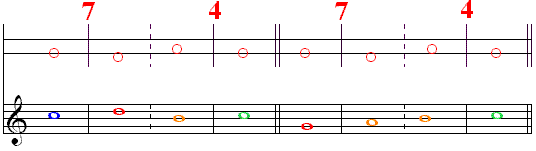In the first example, we find -
Orbit 0, the note C, on the TONIC chord,
Orbit 3, the note D (which becomes Orbit 0, a substitution of Orbit 3), on the COUNTER chord,
Orbit 2, the note B, on the DOMINANT chord, and
Orbit 1, the note C, on the TONIC chord,
in all, the notes C / D \ B / C, a better solution on the M74 than on the M34,
(as the following table will show us).
In the second example, we find -
Orbit 1, the note G (a substitution of Orbit 3), on the TONIC chord,
Orbit 2, the note A (which becomes Orbit 1, a substitution of Orbit 3) on the COUNTER chord,
Orbit 2, the note B, on the DOMINANT chord, and
Orbit 1, the note C, on the TONIC chord,
in all, the notes G / A \ B / C.

Table of Orbit Lines

You will find the following table useful
not only in the quest for harmonic origins when we analyse the Melo-harmony,
but also in the evaluation of the quality of the various options.

 OrbitsChord Pattern 0/3 3 / 2 1 / 2 2 / 1 1 / 1 M6 C / B D / E F / E A / G F / G M7 C / D B / A G / A E / F G / F M3 G / F A / B C / B E / D C / D M4 G / A F / E D / E B / C D / C

In the top line, you will find the fundamental melodic orbit-lines -
Orbit 1 to Orbit 1, Orbit 2 to Orbit 1, Orbit 1 to Orbit 2, Orbit 3 to Orbit 2, and Orbit 0 to Orbit 3.
Note that they are disposed from right to left,
in the order they were generated, starting from the the last cell.
They are now in the order in which time flows (from left to right)
with the most "consequent-oriented" on the right,
and the most "antecedent-oriented" on the left.
In the left column, you will find the four fundamental (nucleus) harmonic cell patterns -
M6, COUNTER / TONIC, M7, TONIC / COUNTER, M3, TONIC / DOMINANT, and M4, DOMINANT / TONIC.
They are symmetrically disposed around the TONIC, and also placed as time flows,
from the antecedent-oriented above to the consequent-oriented below.
In the four-by-five lower-right matrix, you will find the notes produced by the various combinations,
in the key of C major, in which all our songs will be presented.
Since the two notes of each combination are always conjunct (neighbors),
we find here the 14 possible basic combinations (2 for each note),
in bold type, on the right-hand side.
However, two combinations (D / E and F / E ) appear a second time, in light type,
in the Orbit 3 to Orbit 2 line of the M6 and M4 patterns.
This should not surprise us, for we know that there is a "borrowing process"
between the COUNTER and DOMINANT chords,
in which Orbit 3 of one chord is borrowed from Orbit 1 of the other,
more specifically from the Orbit 1 to Orbit 2 line,
as illustrated in the Jordan Diamonds.
Moreover, four more combinations (C / B, C / D, G / F, and G / A) appear a second time, also in light type,
in the Orbit 0 to Orbit 3 procuration line of the four patterns (in the column on the left).
These are the most "antecedent-oriented" Orbit Lines of the whole table,
especially in the top corner,
and the Orbit 1 to Orbit 1 lines, on the right, are the most "consequent-oriented"
especially in the bottom corner.

This table will enable us to retrace the Chord Pattern which generated any given Melody.

Those on a Guided tour should click on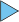in the Navigation Bar below.

Those browsing might wish to see the Entities